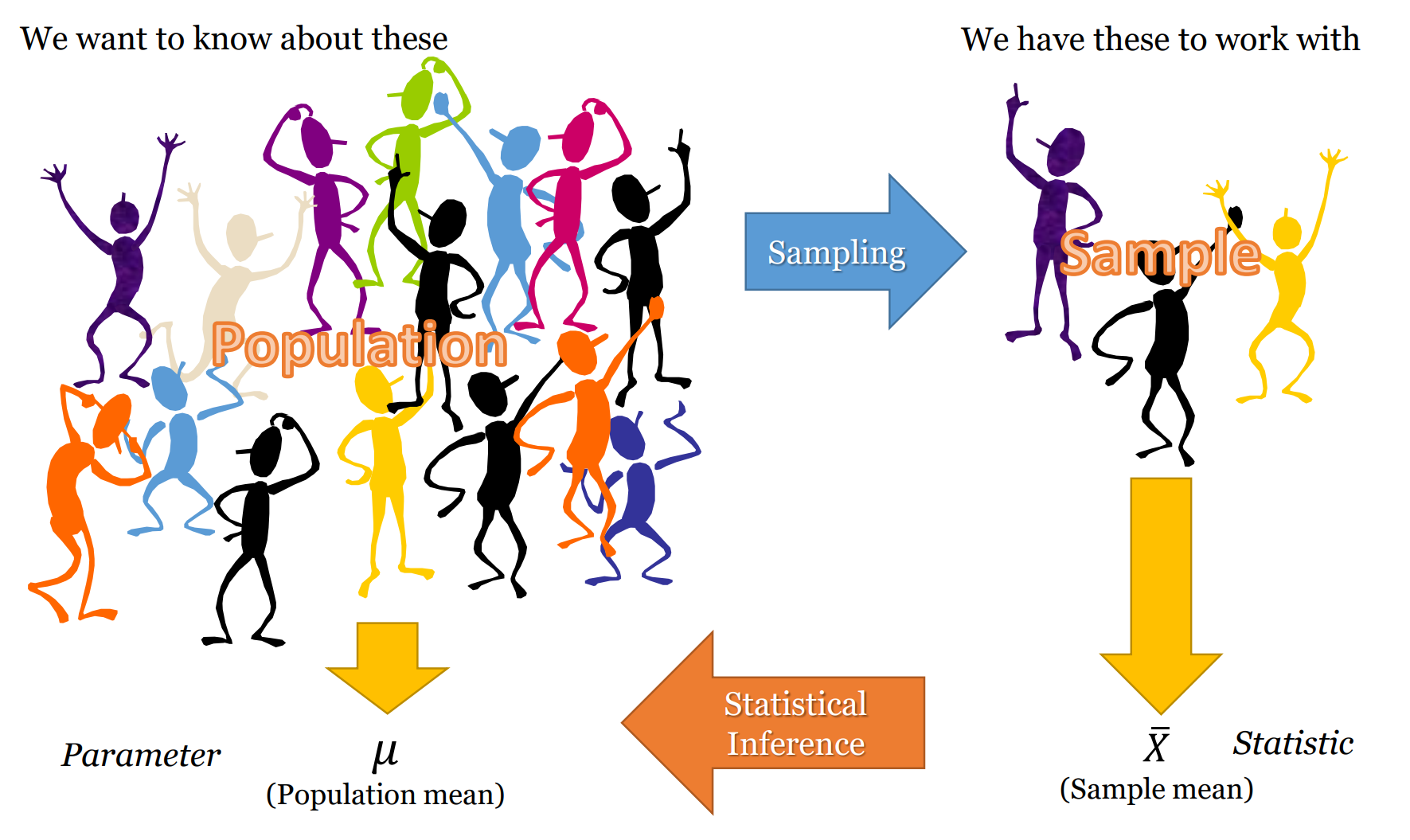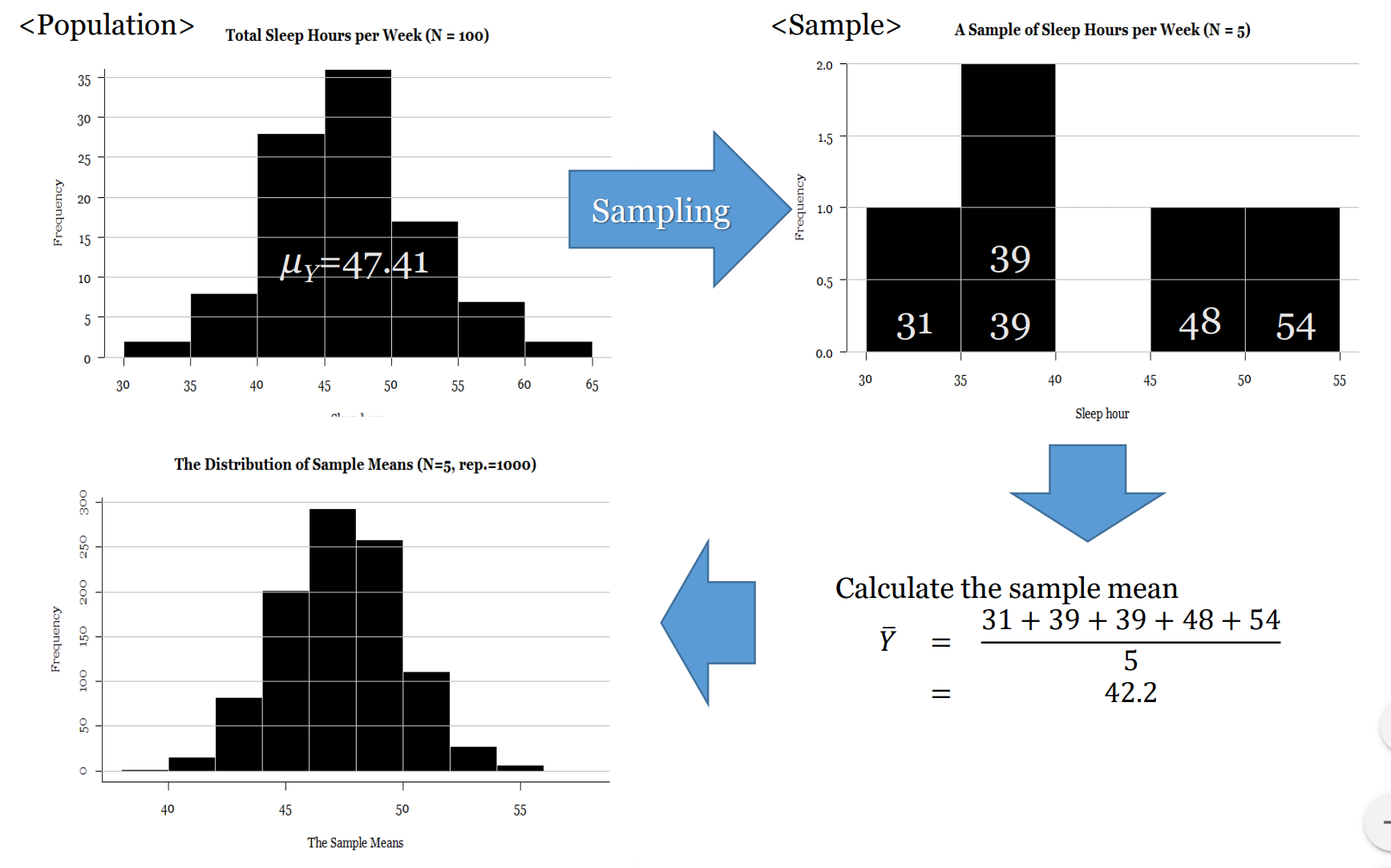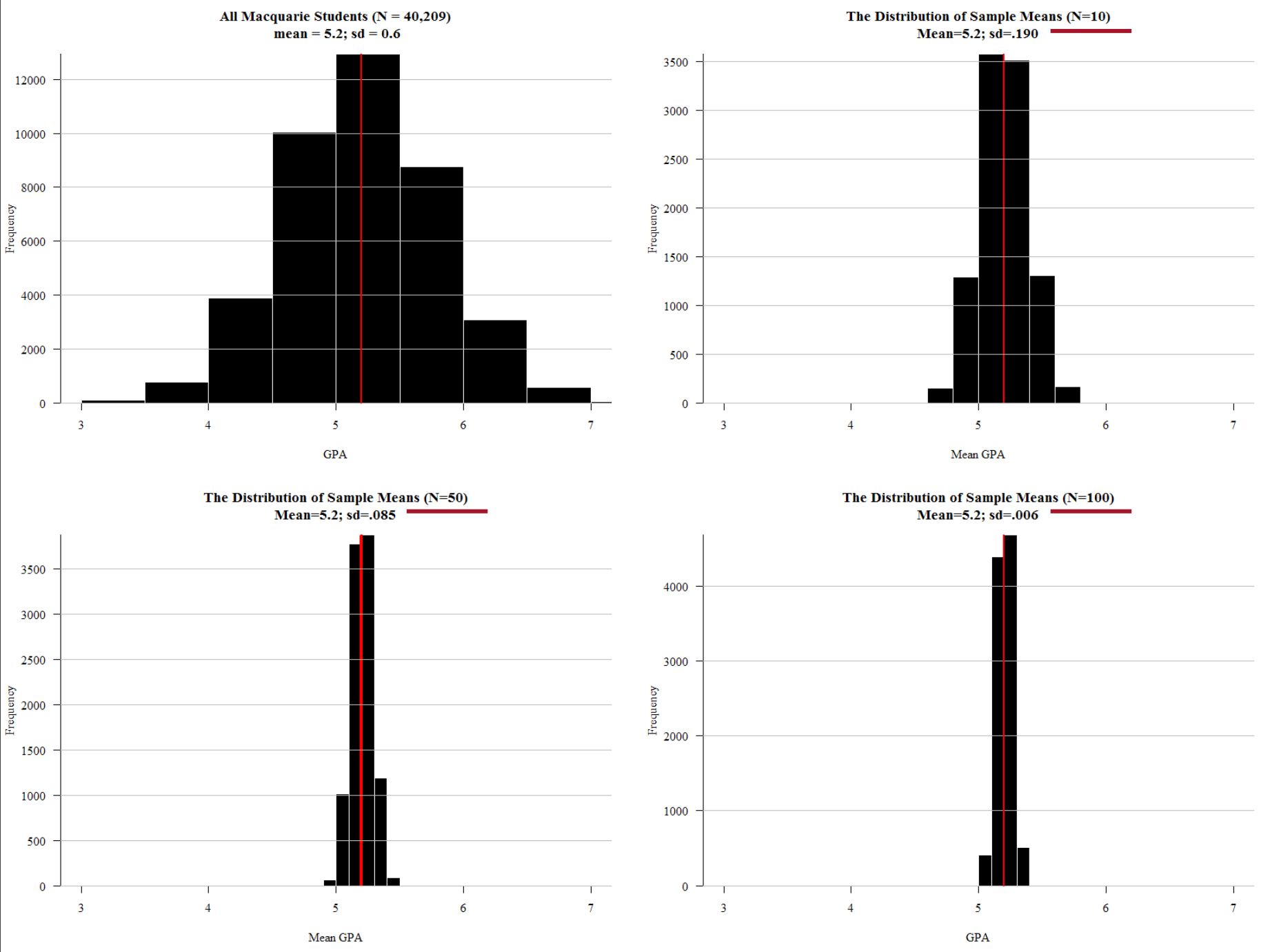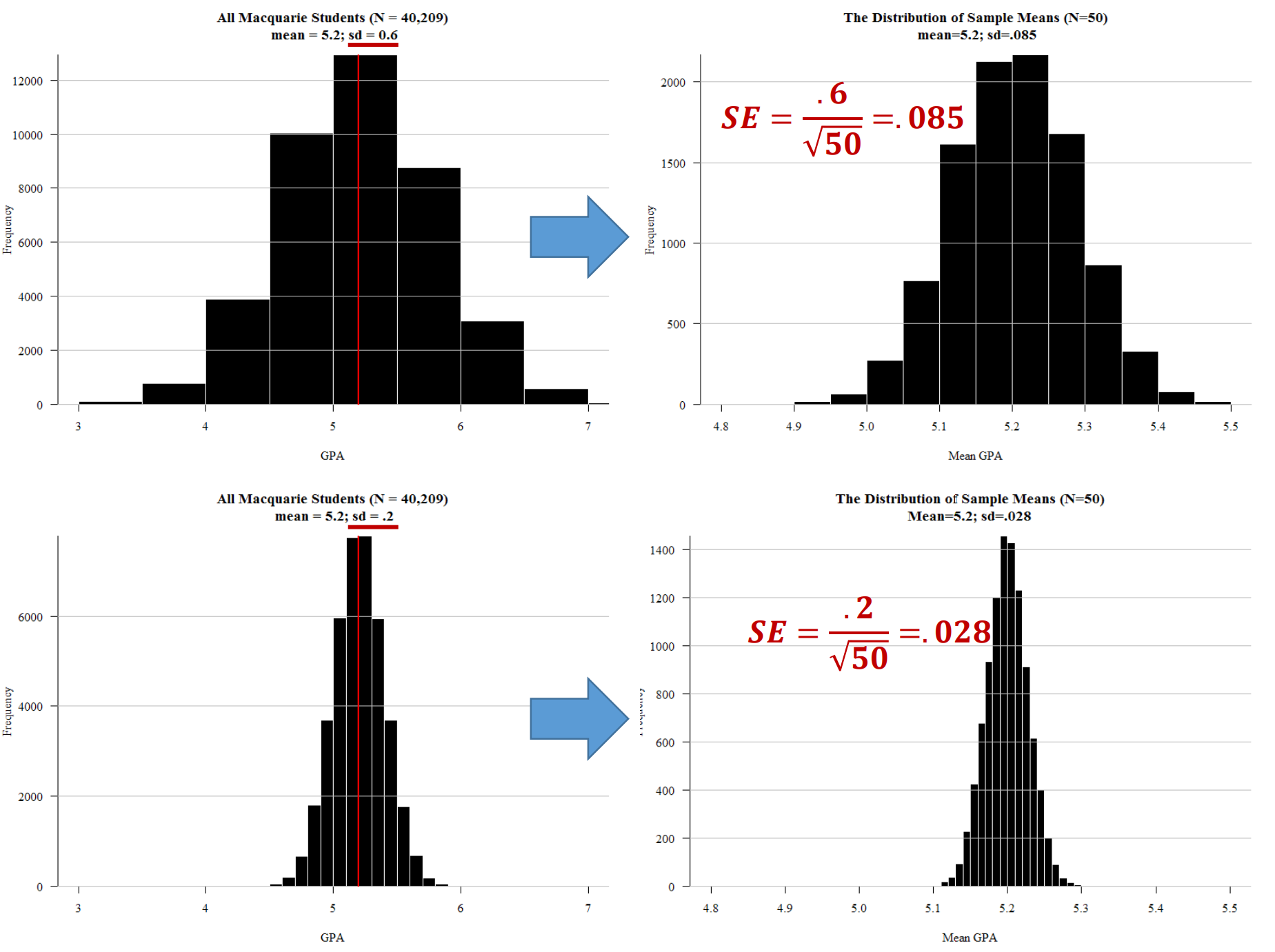# Concepts

Descriptive statistics
Mean/Median/Mode (Central tendency)
Standard deviation/Interquartile range (Variation/dispersion)
Minimum/Maximum
Percentile
N (number of non-missing cases)
Inferential statistics
Population
Population parameter
Sample
Sample statistic
Hypothesis testing
Null hypothesis
Sampling distribution
Central limit theorm
Standard error
Confidence interval
p-value

# Introduction: Two types of statistics

When we use maths for social sciences, we generally use it in one of two ways:

• to generate descriptive statistics - which allow us to summarise a large amount of data in a few numbers - and/or
• to generate inferential statistics - which allow us to draw conclusions about the wider world based on a tiny sample of that world.

# 1. Descriptive statistics: two numbers that are as good as a million

In this section, we will learn about descriptive statistics.

The main purpose of descriptive statistics to summarise a group.

In descriptive statistics, we can actually see, touch, and/or count the group. We use descriptive statistics to describe entire populations we have measured - such as in a census - or to describe a sample (as the sample, not as a representative of the population).

This summary helps us turn a unwheldy set of numbers into one or two: a central tendence, and some indication of variable.

One example of using descriptive statistics is calculating the the median personal weekly personal income of people aged 15 years and over (which is $662) of the 23 million Australian’s who did the 2016 census. We get one number -$662/week - to represent 23,000,000 numbers.

## 1.1 Mean/Median/Mode: The centre

As mentioned, the most common type of descriptive statistics try to summarise the “centre” of a dataset (or more correctly, the centre of the values of a variable). We call this ‘the central tendency’ of the data. The most common example of this is the average - formally called mean - of a set of numbers.

Other measures of the central tendency are the median, and the mode. Box 1 provides definitions, uses, and examples.

All of these measures of the central tendency have a common characteristic: they are trying to find one number that can represent them all. It’s like running a talent contest to find the ‘most ordinary, normal, typical Australian’, except in this case you are trying to find the ‘most ordinary, normal, typical number in this set’.

# Box 1: Definitions, uses, and examples of mean, median, and mode

Mean (the arithmetic mean): The average. The sum of all cases divided by the number of cases.

The mean is good for summarising the centre of a set of numbers that are normally distributed (we will learn about this later). For example, height is normally distributed, so the mean is a good summary measure for a group of people’s heights.

Median: The middle point in a set of numbers. If we order a set of numbers from highest to lowest, and find the number in the middle, this is the median. If two numbers are in the middle (there is an even number of cases), then the median is the average to the two numbers.

The median is good for summarising the centre of a set of numbers that is skewed (i.e. there are a large number of small values, or a large number of large values). For example, income is highly skewed - i.e. unequally distributed, with a large number of poor people, and a few people who are very very rich - and the median is a better measure of this. In Australia the mean income is around $80,000/year, while the median closer to$50,000/year.

Mode: The most common number in a set.

The mode is good for summarising the centre of a set of numbers that are not ordered (i.e. categorical/nominal). For example, if we have a list of 40,000 students and Macquarie Uni, and a variable which is the name of their degree, it doesn’t make sense to talk about the mean or median of the ‘degree’. However the ‘mode’ makes sense. In every day language, we would say the most common degree at Macquarie University is an Arts Degree. Another way to say this, is the ‘mode’ degree is an Arts Degree.

## 1.2 Standard deviation/Interquartile range: Giving variation a number

We know from life, however, that the ‘most ordinary, normal, typical’ single example of anything is a gross simplication.

All sets have variety, and the second major type of descriptive statistic tries to capture this variety, and do it in just one number.

### Standard deviation

The most common measure of variety - or technically ‘variability’, ‘variation’, or ‘dispersion’ - in a set of numbers is probably the standard deviation.

Box 2 provides an explanation of standard deviation - as equation, and also in everyday language.

Intiutively - not strickly - you can think of standard deviation as the average amount - as an absolute value (so negative become positive, and positive stay positive) - that each person’s value for a variable ‘misses’ (deviates) from the mean of the variable.

If the standard deviation is close to zero, then virtually everyone in the dataset has the same value for the variable.

If the standard deviation is large, then you expect that - on average - any particular person you pick from the dataset is going to be quite distant from (greater or lesser than) the mean.

### Interquartile range

While standard deviation is a good measure of variablity for normally distributed data, when we have skewed data (where there are a lot of numbers at one end of the range - like lots of low income people, and a few wealthy in an income distribution), then a better measure of variation is interquartile range, which simply means the range of the ‘middle 50%’ of a dataset. IQR = Q3 - Q1, where Q3 is the number on the boundary between the largest 25%, and the next 25%, and Q1, is the number on the boundary between the smallest 25% and the next 25%.

# Box 2: Standard Deviation: As an equation and in everyday language

\begin{aligned} \text{Standard Deviation of variable x} = &\sqrt{\frac{\sum_{i=1}^n (x_i - \bar x)^2}{N}}\\ \\ \text{Where:} \\ x_i = &\text{value of x for each individual i} \\ \bar x = &\text{ mean of x} \\ N,n = &\text{ number of observations} \\ \sum_{i=1}^n = &\text{sum for all observations from 1 to n} \\ \end{aligned}

While this equation looks scary to many people, I think the best way to break it down - conceptually, and by analogy, not strict mathematically - is to think about this as having just three main parts:

• Variation from mean: First, it basically asks how much, each value of a variable (e.g. the age of each particular student in a class) varies from the mean for the whole set (e.g. the average age of the class). This is represented in the equation as: $(x_i - \bar x)$
• Averaged over all individuals: Second, it takes the average (mean) of this across all observations in the dataset (e.g. all students in the class). This is represented by the sum of (the funny E) divided by N: $\frac{\sum_{i=1}^n \text{variation from mean} }{N}$
• Punishing (counting) big variations more than small ones: Third, it actually squares the deviation from the mean - which has the effect of ‘punishing’ (i.e. inflating or more heavily weighting) larger deviations from the mean more. It then takes the square root of the whole thing, basically to counter act the effect of the squaring, and make it all measured in the same ‘units’ as the mean.

BUT, the take away from all this is: the standard deviation is - more or less - the average amount all values of a variable vary from the mean.

For example, you have two classes of 100 students each, and the average age (mean) of each class is 22, but the standard deviation of the first class is zero, and standard deviation of the second class is one.

Based on this standard deviation, you would know that - roughly and on average - if you picked any, say, six students from each class:

• the six students from the class with zero standard deviation would all be aged 22 (i.e. ages 22, 22, 22, 22, 22, 22), while
• the six students from class with one year standard deviation, would be comprised of students who - on average - deviate from the mean age by one year (e.g. ages 20, 21, 22, 22, 23, 24).
Notice that both groups have the same mean (22), but the second class is much more ‘dispersed’ and ‘varied’: the higher the standard deviation, the more ‘spread out’ the ages in the class are.

## 1.3 Minimum/Maximum: The bounds of our data

Another important set of descriptive statistics of any variable are the minimum and maximum values.

In the world of practical statistics, these numbers are very important because they give a sense of the absolute limits of the values of a dataset.

There are two main reasons you want to know this:

1. Identify errors: Sometimes you make mistakes (or other make mistakes), and the minimum and maximum are often very easy ways to spot this. For example, if the minimum and maximum ages of a sample of adults is 17 and 999, you know that you have problems with your data at both ends. 17 means you have a person who could not consent to do the study, and 999 probably means that you have a missing value, and have not recoded it so the computer/statistical package knows it is missing (and not just a really big number).
2. Identify skewness: It gives you another quick feel for the data. If the distribution is very skewed (e.g. income), then the minimum and maximum will look very different to the minimum and maximum for a classic normal distribution (e.g. height). Apparently if the average wealth was rescaled so it was equal to the average height, then the richest person would be more than 100km tall.

## 1.4 Percentile: For categories

Another type of descriptive statistic is the percentile. For example, the percentage of people who do not speak english at home, or the percentage of people who own dogs.

Percentiles are particularly useful for summarising categorical (nominal) data, as means, standard deviations, minimums, and maximums don’t tend to make sense.

## 1.5 N (number of non-missing cases): Did people actually answer this question?

The final important descriptive statistic we will cover is ‘N’ or ‘n’. This is the number of valid cases in a dataset, or the number of valid cases for a variable.

This number tells you how many people actually answered this question.

This is useful for spotting variables that have large numbers of missing data, which would skew the data, or, again, some sort of data entry problem (such as coding as missing, people who didn’t answer a question, but who can be assumed to be a zero or 1, e.g. “How religious are you?” may not be asked to people without a religion. However, we know that that number is not really missing, it is just very low. How religious are people with out religion? It is pretty safe to assume that they are not very religious!)Figure 1: The Population and The Sample

## 2.1 Population: The big bad world, hidden behind a curtain

As we can see in Figure 1, the sample is drawn from the population, and the sample and the population have their own statistics: the population parameter, and the sample statistic.

### 2.1.1 Population parameter: The number we will never know

Population parameter – A measure (for example, mean or standard deviation) used to describe a population distribution. For example, the mean age of Australians.

## 2.2 Sample: The mini world we can really see and touch

### 2.2.1 Sample statistic: Our approximation of the real world

Sample statistic – A measure (for example, mean or standard deviation) used to describe a sample distribution. For example, the mean age of 500 Australians in a random sample

## 2.3 Hypothesis testing: How do I know if I am wrong?

### 2.3.1 Null hypothesis: The hypothesis that nothing is happening

Research hypothesis (H1) – A statement reflecting the substantive hypothesis. It is always expressed in terms of population parameters, but its specific form varies from test to test. It is also called as alternative hypothesis.

Null hypothesis (H0) – A statement of “no difference (or no relationship),” which contradicts the research hypothesis and is always expressed in terms of population parameters.

Examples:

H1: There is a difference in the maximum height a rabbit and a cat can jump.

• H0: There is no difference in the maximum height a rabbit and a cat can jump..

H1: Sydney has more expensive houses, on average, than Melbourne.

• H0: Sydney has a equal or cheaper houses, on average, than Melbourne.

H1: Law students get higher marks than engineering students in SOCI2000.

• H0: Law students get equal or lower marks, on average, than engineering students in SOCI2000.

### 2.3.2 Sampling distribution: If i could do this survey 1,000 times…Figure 2: A sampling distribution

### 2.3.3 Central limit theorm: If you roll dice infinite times you always get 3.5 (on average)

The central limit theorem says three things:

1. As the number of random samples of size N from a population approaches infinity, the mean of the sampling distribution converges on (approximates) the mean of the population.
2. As the number of random samples from a population gets larger, the sampling distribution approximates a normal distribution
3. As the number of random samples (repetitions -> infinity) of size N (N = sample size for each sample) from a population approaches infinity, the standard deviation of that sampling distribution approximates (the standard deviation of the population/square root of N)

### 2.3.4 Standard error: If I repeated this survey 1,000 times… the answer would vary this much (holds up hands pretending to have caught a big fish).

We call this last number - (the standard deviation of the population/square root of N) - the standard error.Figure 3: The standard error of a sampling distribution gets smaller as the sample size of each sample increases.

Implication: As the sample size gets larger, the standard error (standard deviation of sampling distributions) becomes smaller, which means that a larger sample can better represent the population and thus more accurately infer the parameter.Figure 4: The standard error of a sampling distribution gets smaller as the standard deviation of the population gets smaller.

Implication: As the standard deviation of populations gets smaller, the standard error becomes smaller. When populations have smaller variances, the sample can better represent the population and thus more accurately infer the parameter.

### 2.3.5 Confidence interval: The population parameter is somewhere between here and here

Confidence Level: The likelihood, expressed as a percentage or a probability, that a specified interval will contain the population parameter.

• 95% confidence level (standard) – there is a .95 probability that a specified interval DOES contain the population mean. In other words, there are 5 chances out of 100 (or 1 chance out of 20) that the interval DOES NOT contain the population mean.
• 99% confidence level – there is 1 chance out of 100 that the interval DOES NOT contain the population mean.

Confidence Interval: The range within which we are confident (with a 90%/95%/99% certainty) that the population parameter lies within.

For a normal distribution:

95% Confidence Interval = Sample Statistic +/- 1.96 * Standard Error

Confidence Interval = Sample Statistic +/- Z * Standard Error

### 2.3.6 p-value: the chance I’m wrong. The chance nothing is happening

p-value: The probably of obtaining the test statistic.

• test statistic = ((sample mean) - null_hypothesis)/standard error

Since null_hypothesis is generally that nothing is happening, i.e. 0, then test statistic reduces to:

• test statistic = sample_statistic/standard_error

Main examples of test statistics = Z, t.

• t is for samples n < 81
• z is for everything else.

p-value for various levels of z-score:

• z = 1.65 p = .10
• z = 1.96 p = .05
• z = 2.33 p = .01
Last updated on 04 May, 2020 by Dr Nicholas Harrigan (nicholas.harrigan@mq.edu.au)